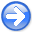PreconditioningNext: SeaBeam Up: Preconditioning Previous: INVERSE LINEAR INTERPOLATION

# EMPTY BINS AND PRECONDITIONING

There are at least three ways to fill empty bins. Two require a roughening operatorwhile the third requires a smoothing operator, which (for comparison purposes) we denote. The three methods are generally equivalent though they differ in significant details.

The original way in Chapteris to restore missing data by ensuring the restored data, after specified filtering, has minimum energy, say. Introduce the selection mask operator, a diagonal matrix with ones on the known data and zeros elsewhere (on the missing data). Thus,or:(43)

where we defineto be the data with missing values set to zero by.

A second way to find missing data is with the set of goals:(44)

and take the limit as the scalar. At that limit, we should have the same result as equation (43).

There is an important philosophical difference between the first method and the second. The first method strictly honors the known data. The second method acknowledges that when data misfits the regularization theory, it might be the fault of the data, so the data need not be strictly honored. Just what balance is proper falls to the numerical choice of, a nontrivial topic.

A third way to find missing data is to precondition equation (44), namely, try the substitution.(45)

There is no simple way of knowing beforehand what is the best value of. Practitioners like to see solutions for various values of. Of course, that can cost a lot of computational effort. Practical exploratory data analysis is more pragmatic. Without a simple clear theoretical basis, analysts generally begin fromand abandon the fitting goal. Implicitly, they take. Then, they examine the solution as a function of iteration, imagining that the solution at larger iterations corresponds to smaller. There is an eigenvector analysis indicating some kind of basis for this approach, but I believe there is no firm guidance.

SubsectionsPreconditioningNext: SeaBeam Up: Preconditioning Previous: INVERSE LINEAR INTERPOLATION

2015-05-07